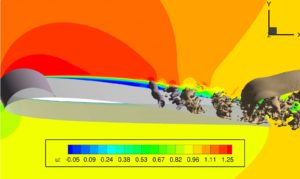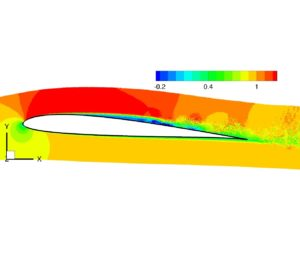# Fluid Mechanics

## Fluid Mechanics (2L + 1E) [B.Sc. General Engineering Science]

Fluid flows play an important role in nature as well as nearly all technical applications. It leads to the transport of mass, momentum and energy and thus dominates a variety of significant processes. Breathing of air or blood flow in veins and arteries are only two examples relevant for us every second of our life. The list of examples in engineering sciences is endless starting from obvious cases such as the flow around a wing generating lift forces or less obvious cases for example the pressurization and air conditioning of the airplane cabin to stay in the context of aircrafts.

In this introductory course fundamentals of fluid dynamics are discussed which are relevant for the description, the analysis and the prediction of different fluid flow problems. Relying on the continuum mechanics approach, all fluid flow problems can be expressed by conservation equations of mass, momentum and energy. Thus, it of utmost importance to understand this set of equations and how they can be applied to solve fluid mechanical problems of practical relevance. This course is a first step into the wonderful and fascinating world of fluid mechanics. (Follow-up graduate courses are listed below).

### Objectives

Participants of the course on Fluid Mechanics will learn to:

• understand the fundamentals of fluid mechanics and its importance for a variety of technical applications
• read and understand the governing equations of fluid mechanics and its boundary conditions
• solve classical fluid mechanical problems based on simplified governing equations analytically
• gain a deep understanding of fluid mechanics and its underlying principles

### Content

1. Importance of fluid mechanics
2. Continuum mechanics approach and conservation principle of mass and momentum
3. Navier-Stokes equations and boundary conditions
4. Hydrostatics and aerostatics (theory and applications)
5. Hydrodynamics (theory and applications)
6. Integral form of the governing equations (conservation of  mass and momentum) including applications
7. Similarity theory and dimensional analysis
8. Steady fluid flows of incompressible viscous fluids

### Teaching and learning methods

In the lectures some demonstration experiments are shown to support the theoretical considerations. The exercises are organized in small study groups, in which the students have to solve fluid mechanical problems themselves supported by the research assistants.• Slides
• Exercises
• Formulary
• Exams

### Follow-up graduate courses (in German)

Letzte Änderung: 25. März 2019